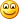# Code for drawing a line using Bresenham algorithm in Javascript

Here is the snippet for drawing a line in javascript.

function drawline(xa,ya,xb,yb){
var dx=Math.abs(xa-xb);
var dy=Math.abs(ya-yb);
var p=2*dy-dx;
var twody=2*dy;
var twodydx=2*(dy-dx);
var x,y,xend;

if(xa>xb)
{
x=xb;
y=yb;
xend=xa;
}
else
{
x=xa;
y=yb;
xend=xb;
}
drawpix(x,y);
while(x<xend)
{
x++;
if(p<0)
{
p+=twody;
}
else
{
y++;
p+=twodydx;
}
drawpix(x,y);
}
}

As in previous blog you can find the code for pixel drawing code. Here is the link for the pixel drawing code. http://bit.ly/Wzm0xG

Go through it and happy coding…Share

## 4 thoughts on “Code for drawing a line using Bresenham algorithm in Javascript”

1.Hi there! Your code have a mistake:

if(xa>xb)
{
x=xb;
y=yb;
xend=xa;
}
else
{
x=xa;
y=yb; <<<<<<<<<<<<<< This should be ya
xend=xb;
}

And thanks for sharing this code, once corrected works like a charm 😀

1.Thank you for correcting me. 🙂 i will make sure that next time the code works

2.I need your help..how to implement this using canvas

1.You need to use this algirthm in javascript. and link it with canvass. 🙂